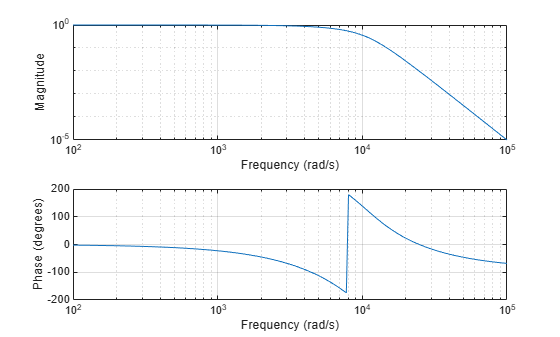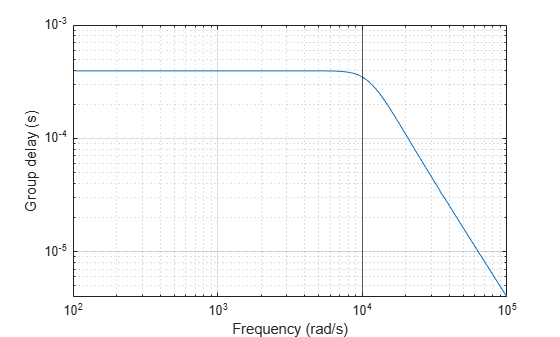# Frequency Response of Lowpass Bessel Filter

Design a fifth-order analog lowpass Bessel filter with approximately constant group delay up to $1{0}^{4}$ rad/second. Plot the magnitude and phase responses of the filter using `freqs`.

```[b,a] = besself(5,10000); freqs(b,a)```Compute the group delay response of the filter as the derivative of the unwrapped phase response. Plot the group delay to verify that it is approximately constant up to the cutoff frequency.

```[h,w] = freqs(b,a,1000); grpdel = diff(unwrap(angle(h)))./diff(w); clf semilogx(w(2:end),grpdel) xlabel('Frequency (rad/s)') ylabel('Group delay (s)')```